Open In App
Related Articles
• Write an Interview Experience

# Introduction to Matplotlib

Matplotlib is an amazing visualization library in Python for 2D plots of arrays. Matplotlib is a multi-platform data visualization library built on NumPy arrays and designed to work with the broader SciPy stack. It was introduced by John Hunter in the year 2002. One of the greatest benefits of visualization is that it allows us visual access to huge amounts of data in easily digestible visuals. Matplotlib consists of several plots like line, bar, scatter, histogram etc.

Installation: Windows, Linux and macOS distributions have matplotlib and most of its dependencies as wheel packages. Run the following command to install matplotlib package. But before that make sure Python and PIP are already installed on a system :

To install pip you can refer to this article : Python PIP

After checking Python and PIP in your system, You need to run this command to install Matplotlib.

`python -mpip install -U matplotlib`

### Importing matplotlib

After successfully installing matplotlib , You can run this command to import matplotlib on your system.

`Import matplotlib`

## Basic plots in Matplotlib

Matplotlib comes with a wide variety of plots. Plots help to understand trends, and patterns, and to make correlations. They’re typically instruments for reasoning about quantitative information. Some of the sample plots are covered here.

## Line plot using Matplotlib

By importing the matplotlib module, defines x and y values for a plots, plots the data using the plot() function and it helps to display the plot by using the show() function . The plot() creates a line plot by connecting the points defined by x and y values.

## Python3

 `# importing matplotlib module``from` `matplotlib ``import` `pyplot as plt``# x-axis values``x ``=` `[``5``, ``2``, ``9``, ``4``, ``7``]``# Y-axis values``y ``=` `[``10``, ``5``, ``8``, ``4``, ``2``]``# Function to plot``plt.plot(x,y)``# function to show the plot``plt.show()`

Output :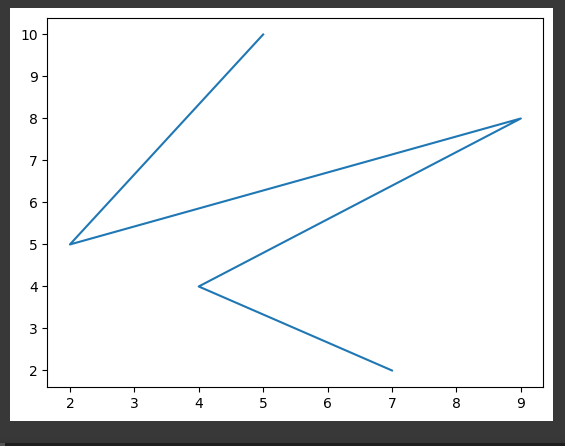Plots in Matplotlib

## Bar plot using Matplotlib

By using matplotlib library in python , it allows us to access the functions and classes provided by the library for plotting. There are tow list x and y are defined . This function creates a bar plot by taking x-axis and y-axis values as arguments and generates the bar plot based on those values.

## Python3

 `# importing matplotlib module``from` `matplotlib ``import` `pyplot as plt``# x-axis values``x ``=` `[``5``, ``2``, ``9``, ``4``, ``7``]``# Y-axis values``y ``=` `[``10``, ``5``, ``8``, ``4``, ``2``]``# Function to plot the bar``plt.bar(x,y)``# function to show the plot``plt.show()`

Output: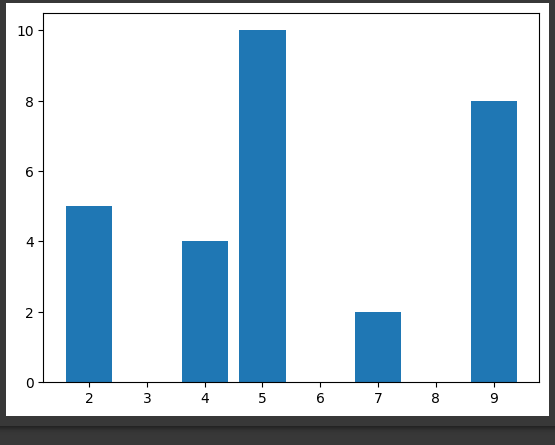Bar Plot

## Histogram using Matplotlib

By using the matplotlib module defines the y-axis values for a histogram plot. Plots in histogram using the hist() function and displays the plot using the show() function. The hist() function creates a histogram plot based on the values in the y-axis list.

## Python3

 `# importing matplotlib module``from` `matplotlib ``import` `pyplot as plt``# Y-axis values``y ``=` `[``10``, ``5``, ``8``, ``4``, ``2``]``# Function to plot histogram``plt.hist(y)``# Function to show the plot``plt.show()`

Output: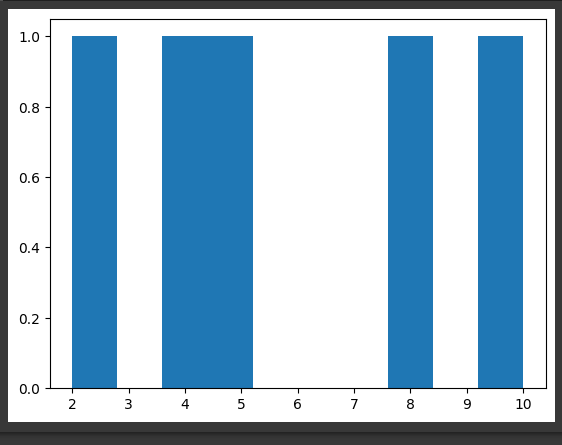Histogram

## Scatter Plot using Matplotlib

By imports the matplotlib module, defines x and y values for a scatter plot, plots the data using the scatter() function, and displays the plot using the show() function. The scatter() function creates a scatter plot by plotting individual data points defined by the x and y values.

## Python3

 `# importing matplotlib module``from` `matplotlib ``import` `pyplot as plt``# x-axis values``x ``=` `[``5``, ``2``, ``9``, ``4``, ``7``]``# Y-axis values``y ``=` `[``10``, ``5``, ``8``, ``4``, ``2``]``# Function to plot scatter``plt.scatter(x, y)``# function to show the plot``plt.show()`

Output :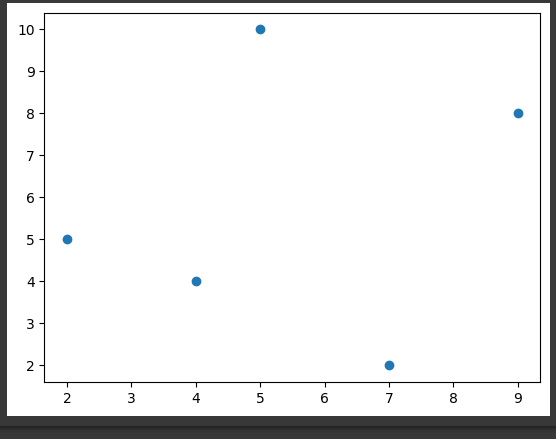Scatter Plot

We can also plot a scatter plot using the plot() method by providing the marker style as ‘o’.

## Python3

 `# importing matplotlib module``from` `matplotlib ``import` `pyplot as plt``# x-axis values``x ``=` `[``5``, ``2``, ``9``, ``4``, ``7``]``# Y-axis values``y ``=` `[``10``, ``5``, ``8``, ``4``, ``2``]``# Function to plot scatter using plot() method``plt.plot(x,y,``'o'``)``# function to show the plot``plt.show()`

Output: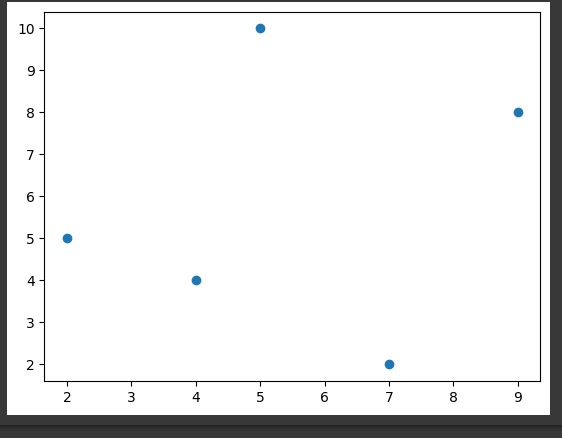Scatter Plot by using plot()